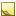﻿Volume data typeVolume data type

The data type Volume a volume measurement.

The TDE data types for quantifiable data (numeric values with units of measure) are all stored as double and are handled similarly for template scripting.Tip

Since every quantifiable data type is stored as double, be sure to account for that when you test equivalency (for example, 1 may actually be stored as 0.999999). Either round off the values being compared, or use ranges for the comparison.Units
• liter or L — default

• cubic meter or m^3

• cubic foot or ft^3

• US gallon or gal

• US fluid ounce or fl oz

• US quart or qt

• US pint or pt

• imperial gallon or imp gal

• milliliter or ml

• cubic inch or in3

Data from TDE defaults to the first unit listed for each data type. In the [Output] section, you can convert these values to the units you need:

```[Output]
TripFuelQuarts(qt) = input.TripFuelUsed   # converts liters to quarts```Field length

The effective range of this data type is: 0 to 1,000,000,000 LJavaScript conversion

When you use the optional [Script] section to insert your own JavaScript functions, access this data type as follows:

Constructor
JavaScript
`new Volume({Unit:Value})`
Available fields for (get,set)

You can access the valid units of measure as fields:

• .liter

• ."US gallon"

• ."US fluid ounce"

• ."US quart"

• ."US pint"

• ."imperial gallon"

• .milliliter

• ."cubic inch"Tip

Use square brackets and double quotes around TDE units of measure that contain spaces. For example: row.TotalFuelUsed["US gallon"]. Otherwise, you can use dot notation, such as row.TotalFuelUsed.milliliter.